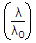# Electronics and Communication Engineering - Microwave Communication

### Exercise :: Microwave Communication - Section 5

16.

The usable bandwidth of ridge wave guide

 A. is lower than that of rectangular waveguide B. is higher than that of rectangular waveguide C. is almost same as that of rectangular waveguide D. is very small as compared to that of rectangular waveguide

Answer: Option B

Explanation:

No answer description available for this question. Let us discuss.

17.

Assertion (A): In TE mode for rectangular waveguide.

Reason (R): In TM mode for rectangular waveguide.

 A. Both A and R are correct and R is correct explanation of A B. Both A and R are correct but R is not correct explanation of A C. A is correct but R is wrong D. A is wrong but R is correct

Answer: Option B

Explanation:

No answer description available for this question. Let us discuss.

18.

The attenuation characteristics of a loaded line is similar to that of

 A. low pass filter B. high pass filter C. band pass filter D. band stop filter

Answer: Option D

Explanation:

No answer description available for this question. Let us discuss.

19.

A microstrip line has ∈r = 9. The normalised wavelengthis about

 A. 10 B. 2 C. 1 D. 0.4

Answer: Option D

Explanation:

No answer description available for this question. Let us discuss.

20.

If the repeller voltage in a klystron oscillator is increased the transit time of electron

 A. will be increased B. will be decreased C. will remain the same D. either (a) or (b)

Answer: Option C

Explanation:

No answer description available for this question. Let us discuss.

#### Current Affairs 2021

Interview Questions and Answers